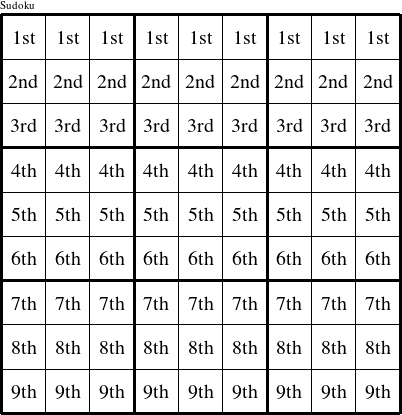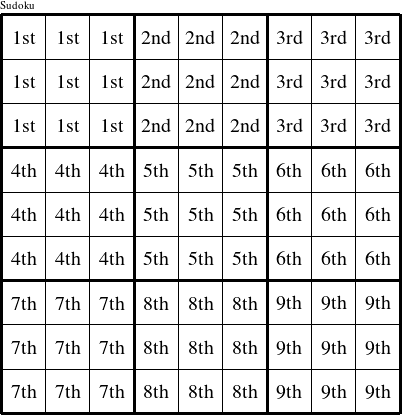#### Printable version

Detailed instructions for working a group puzzle of type 'Sudoku'

The 9 elements in each group of this type of puzzle are: 1, 2, 3, 4, 5, 6, 7, 8, and 9.

Every group in the puzzle must contain exactly this set of elements.

####In a Sudoku puzzle, each row is a group numbered as shown in the figure above.

####In a Sudoku puzzle, each column is a group numbered as shown in the figure above.

####In a Sudoku puzzle, each 3x3 square is a group numbered as shown in the figure above.